# How to Use axis=0 and axis=1 in Pandas?

• Difficulty Level : Easy
• Last Updated : 19 Dec, 2021

In this article, we will discuss how to use axis=0 and axis=1 in pandas using Python.

Sometimes we need to do operations only on rows, and sometimes only on columns, in such situations, we specify the axis parameter. In this article, let’s see a few examples to know when and how to use the axis parameter. In pandas axis = 0 refers to horizontal axis or rows and axis = 1 refers to vertical axis or columns.

## AXIS =0

When the axis is set to zero while performing a specific action, the action is performed on rows that satisfy the condition.

Dataset Used: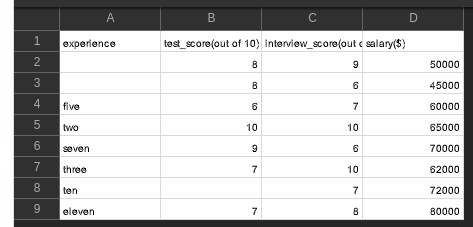Example: Using axis=0

## Python3

 `# importing packages ` `import` `pandas as pd ` ` `  `# importing our dataset ` `df ``=` `pd.read_csv(``'hiring.csv'``) ` ` `  `# dropping the column named 'experience' ` `df ``=` `df.drop([``0``, ``3``], axis``=``0``) ` ` `  `# 'viewing the dataframe ` `df.head() `

Output: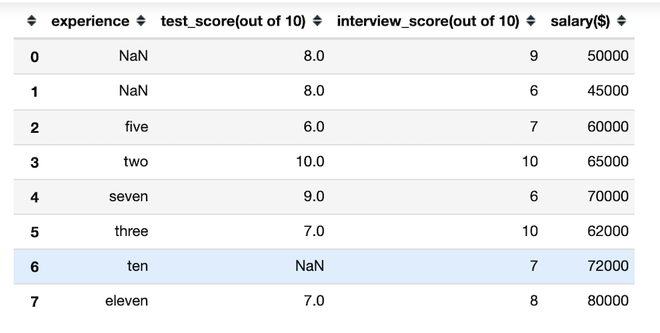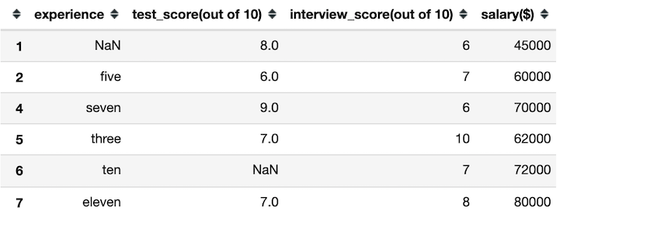Example: Using axis=0

## Python3

 `# importing packages ` `import` `pandas as pd ` ` `  `# creating a dataset ` `df ``=` `pd.DataFrame([[``1``, ``2``, ``3``], [``4``, ``5``, ``6``], ` `                   ``[``7``, ``8``, ``9``], [``10``, ``11``, ``12``]], ` `                  ``columns``=``[``'a'``, ``'b'``, ``'c'``]) ` ` `  `# viewing the dataFrame ` `print``(df) ` ` `  `# finding mean by rows ` `df.mean(axis``=``'rows'``) `

Output: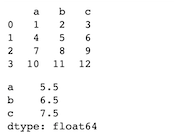## AXIS=1

When the axis is set to one while performing a specific action, the action is performed on column(s) that satisfy the condition.

Example: Using axis=1

## Python3

 `# importing packages ` `import` `pandas as pd ` ` `  `# importing our dataset ` `df ``=` `pd.read_csv(``'hiring.csv'``) ` ` `  `# dropping the column named 'experience' ` `df ``=` `df.drop([``'experience'``], axis``=``1``) ` ` `  `# 'viewing the dataframe ` `df.head() `

Output: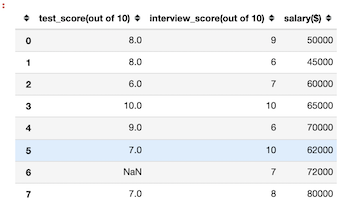Example: Using axis=1

## Python3

 `# importing packages ` `import` `pandas as pd ` ` `  `# creating a dataset ` `df ``=` `pd.DataFrame([[``1``, ``2``, ``3``], [``4``, ``5``, ``6``], ` `                   ``[``7``, ``8``, ``9``], [``10``, ``11``, ``12``]],  ` `                  ``columns``=``[``'a'``, ``'b'``, ``'c'``]) ` ` `  `# viewing the dataFrame ` `print``(df) ` ` `  `# finding mean by columns ` `df.mean(axis``=``'columns'``) `

Output: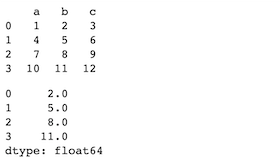My Personal Notes arrow_drop_up
Recommended Articles
Page :